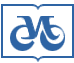## Издательство СО РАН

Адрес Издательства СО РАН: Россия, 630090, а/я 187
Новосибирск, Морской пр., 2# Главная – Журналы – Журнал структурной химии 2010 номер 5

## Журнал структурной химии

### 2010 год, номер 5

#### Prediction of water-to-polydimethylsiloxane partition coefficient for some organic compounds using QSPR approaches

H. Golmohammadi1, Z. Dashtbozorgi2
1 Department of Chemistry, Mazandaran University, Babolsar, Iran, hassan.gol@gmail.com
2 Department of Chemistry, Islamic Azad University, Science and Research Branch, Tehran, Iran
Ключевые слова: quantitative structure-property relationship, water-to-polydimethylsiloxane partition coefficient, artificial neural network, multiple linear regression, genetic algorithm
Страницы: 870-882

Аннотация

A Quantitative Structure - Property Relationship (QSPR) model based on Genetic Algorithm (GA), Multiple Linear Regression (MLR) and Artificial Neural Network (ANN) techniques was developed for the prediction of water-to-polydimethylsiloxane partition coefficients (log KPDMS-water) of 139 organic compounds. A suitable set of molecular descriptors was calculated and important descriptors were selected by genetic algorithm and stepwise multiple regression. These descriptors were: Minimum Atomic Orbital Electronic Population (Pμμ), Kier Shape Index (order 3) (3κ), Polarity Parameter / Square Distance (PP), and Complementary Information Content (order 2) (2CIC). In order to find a better way to depict the nonlinear nature of the relationships, these descriptors were used as inputs for a generated ANN. The root mean square errors for the neural network calculated log KPDMS-water of training, test, and validation sets were 0.116, 0.179, and 0.183, respectively, which are smaller than those obtained by MLR model (0.422, 0.425, and 0.480, respectively). The results obtained showed the ability of developed artificial neural network to predict water-to-polydimethylsiloxane partition coefficients of various organic compounds. Also, the results revealed the superiority of the artificial neural network over the multiple linear regression model.